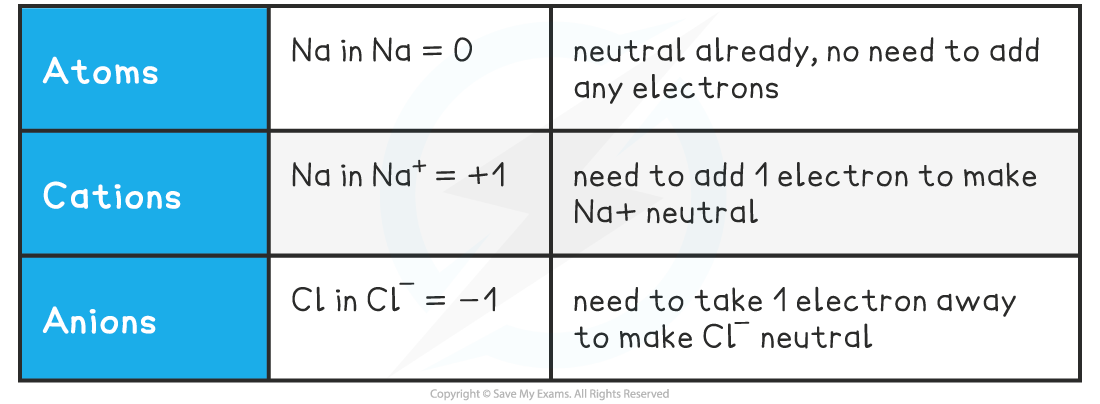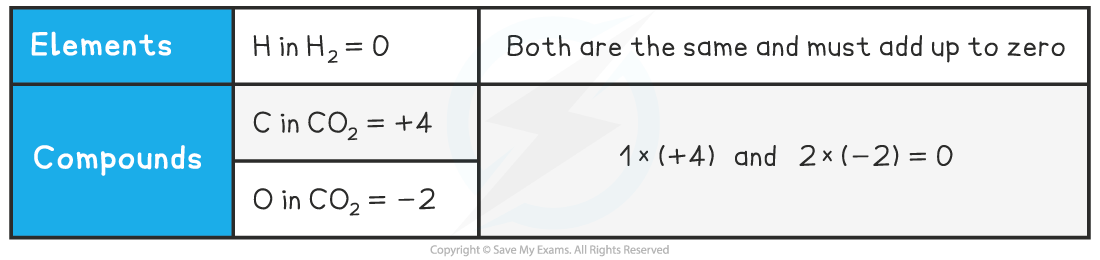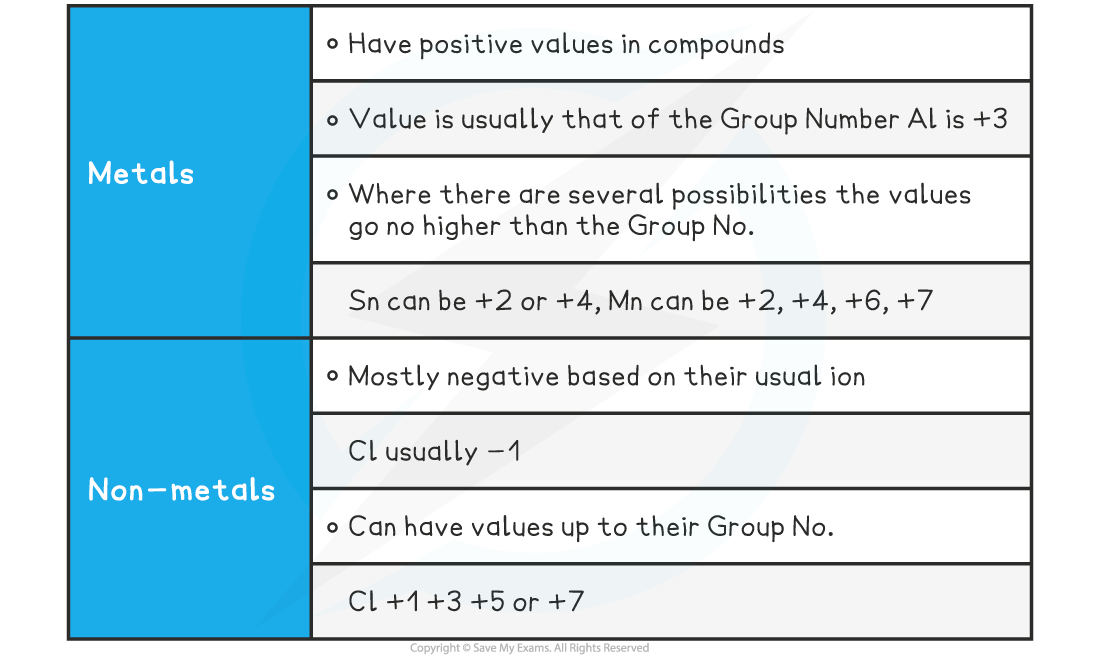# Edexcel A Level Chemistry:复习笔记2.1.1 Oxidation Number

### Defining Oxidation Number

• There are three definitions of oxidation and reduction  used in different branches of chemistry
• Oxidation and reduction can be used to describe any of the following processes

Definitions and Examples of Oxidation & ReductionUse the acronym "Oil Rig" to help you remember the definitions of oxidation and reduction

#### Oxidation Number

• The oxidation number of an atom is the charge that would exist on an individual atom if the bonding were completely ionic
• It is like the electronic ‘status’ of an element
• Oxidation numbers are used to
• Tell if oxidation or reduction has taken place
• Work out what has been oxidised and/or reduced
• Construct half equations and balance redox equations

Oxidation Numbers of Simple Ions#### Worked Example

What are the oxidation numbers of the elements in the following species?

a) C                b)  Fe3+                       c)  Fe2+

d) O2-             e)  He                          f)  Al3+

a) 0     b) +3    c) +2

d) -2    e) 0      f) +3

• So, in simple ions, the oxidation numbers of the atom is the charge on the ion:
• Na+, K+, H+ all have an oxidation number of +1
• Mg2+, Ca2+, Pb2+ all have an oxidation number of +2
• Cl–, Br–, I– all have an oxidation number of -1
• O2-, S2- all have an oxidation number of -2

### Calculating Oxidation Numbers

#### Oxidation Number Rules

• A few simple rules help guide you through the process of determining the oxidation number of any element
• Remember, you are determining the oxidation number of a single atom
• The oxidation number (ox.no.) refers to a single atom in a compound

Oxidation Number Rules Table#### Molecules or Compounds

• In molecules or compounds, the sum of the oxidation numbers on the atoms is zero

Oxidation Number in Molecules or Compounds• Because CO2 is a neutral molecule, the sum of the oxidation numbers must be zero
• For this, one element must have a positive oxidation number and the other must be negative

How do you determine which is the positive one?

• The more electronegative species will have the negative value
• Electronegativity increases across a period and decreases down a group
• O is further to the right than C in the periodic table so it has the negative value

How do you determine the value of an element’s oxidation number?

• From its position in the periodic table and / or
• The other element(s) present in the formula
• The oxidation numbers of all other atoms in their compounds can vary
• By following the oxidation number rules, the oxidation number of any atom in a compound or ion can be deduced
• The position of an element in the periodic table can act as a guide to the oxidation number

Oxidation Numbers & the Periodic Table